Function Repository Resource:

# CanonicalBasis

Obtain the canonical bases for selected spaces of matrices and functions

Contributed by: Dennis M Schneider
 ResourceFunction["CanonicalBasis"][type,var,degree] returns the canonical basis for polynomials or trigonometric polynomials of degree at most degree in the variable var. ResourceFunction["CanonicalBasis"][type,size] returns the canonical basis for the space of matrices of type type and size size.

## Details and Options

The basis returned by ResourceFunction["CanonicalBasis"] is a basis for the given vector space over the real numbers, not the complex numbers.
The value for type can be any of the following:
 "DiagonalMatrices" "HermitianMatrices" "Matrices" "Polynomials" "SkewHermitianMatrices" "SkewSymmetricMatrices" "SymmetricMatrices" "TraceZeroMatrices" "TrigonometricPolynomials"

## Examples

### Basic Examples (2)

The canonical basis for the space of polynomials in x of degree 5 or less:

 In:=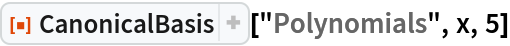Out=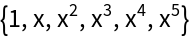The canonical basis for the space of 2×3 matrices:

 In:=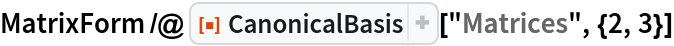Out=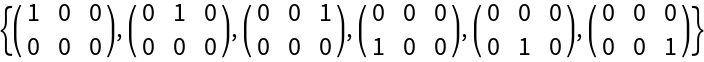### Scope (6)

#### Function spaces (1)

The canonical basis for the space of trigonometric polynomials in x of degree 5 or less:

 In:=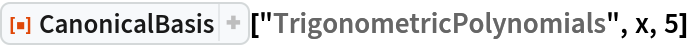Out=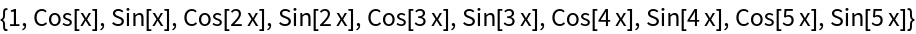#### Matrix spaces (5)

The canonical basis for the space of 3×3 square matrices:

 In:=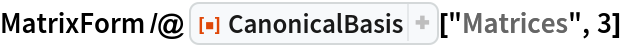Out=The canonical basis for the space of 3×3 symmetric matrices:

 In:=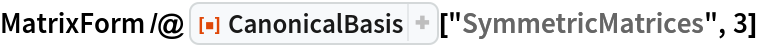Out=The canonical basis for the space of 3×3 skew-symmetric matrices:

 In:=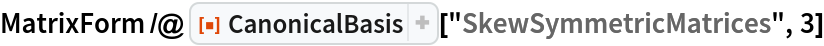Out=The Hermitian matrices of given degree do not form a vector space over the complex numbers, but they do form a vector space over the real numbers:

 In:=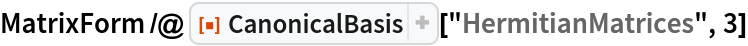Out=As with Hermitian matrices, the skew-Hermitian matrices of given degree form a vector space over the real numbers:

 In:=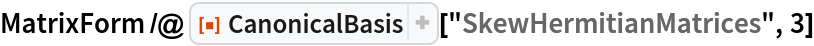Out=### Applications (2)

A Manipulate illustrating an array of function-related examples:

 In:=Out=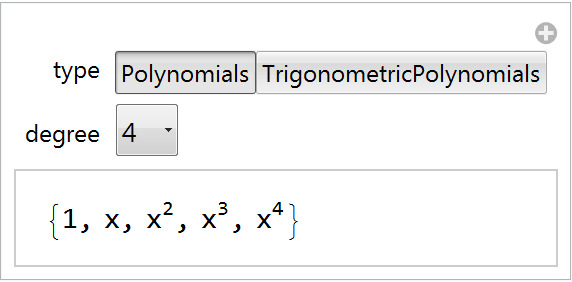A Manipulate illustrating an array of matrix-theoretic examples:

 In:=Out=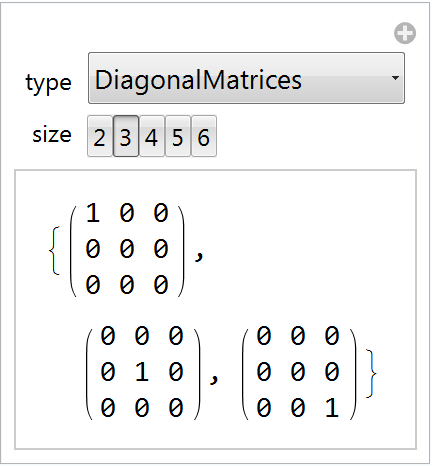## Publisher

Dennis M Schneider

## Version History

• 1.0.0 – 04 October 2019